# 【Unity3D开发小游戏】2D乒乓球游戏

## 二、效果图：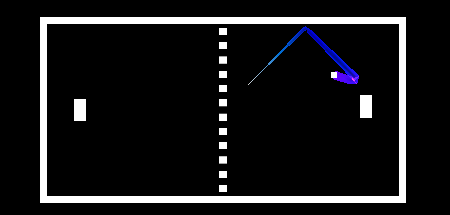## 三、正文

### 游戏规则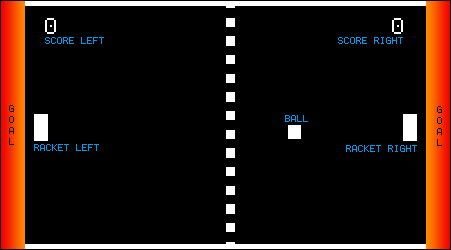游戏规则应该非常类似于原来的乒乓球。如果球击中左边的墙，右边的球员得分一分。如果球击中右面墙，左边的球员就得分。如果它撞到了顶部或底部的墙壁，那么它就会反弹。每个球员将有一个球拍，可以上下移动，以击退球。

• 如果球拍击中球顶拐角处，它应该会向我们的最高边界反弹。
• 如果球拍击中球中心，然后它应该反弹到右边，而不是向上或向下。
• 如果球拍击中球底部拐角处，它应该会反弹到我们的底部边界。

## 造墙

### 墙壁的物理效果

Unity伴随着一个非常强大的物理引擎，我们所要做的就是告诉Unity，我们的墙壁应该是Colliders，我们在Hierarchy选择它们：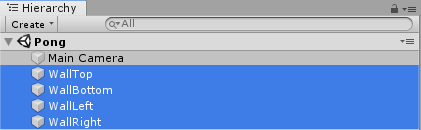之后，我们单击Add Component按钮中的Inspector然后选择Physics 2D -> Box Collider 2D，或者直接搜索Box，如下图：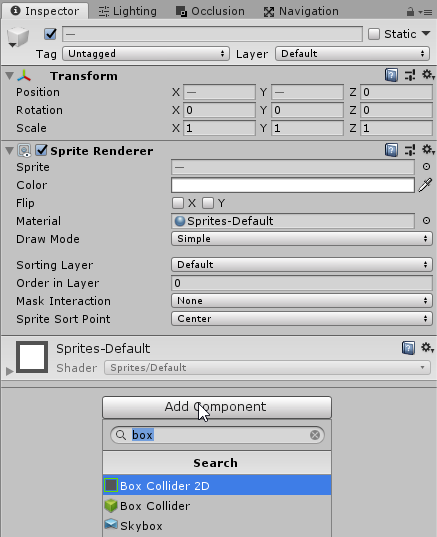注意：无论我们在Inspector中做什么，都将对Hierarchy中选择的所有对象执行。因为我们选择了所有的四面墙，他们现在都有了Collider 。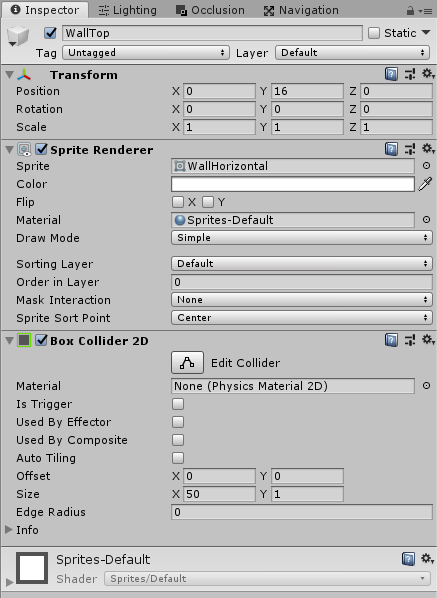### 创造球拍

Racket.png 注意：右击图像，选择但作为.。并将其保存在项目的资产文件夹。 我们将使用以下方法导入设置为此：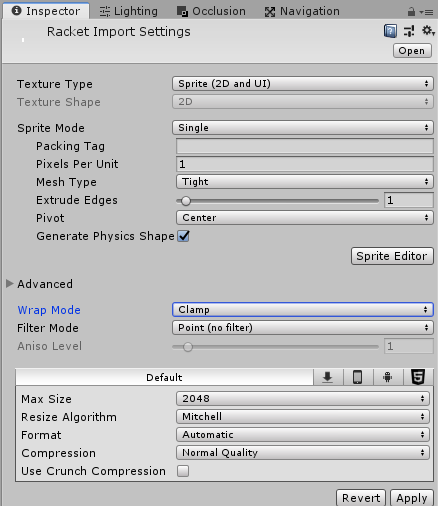我们的比赛将有两个球员，一个在左边，一个在右边。所以让我们把球拍拖到游戏里两次，然后把它放在左边和右边：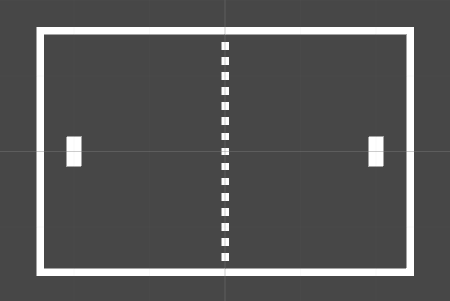### 球拍的Physics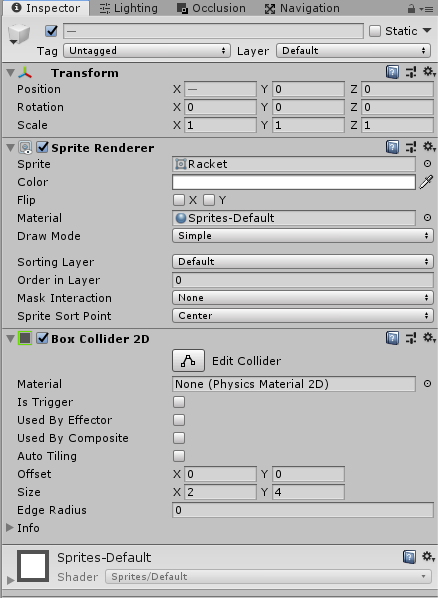球员也应该能够将球拍向上和向下移动。但是当球拍与墙相撞时，应该停止向上移动(或向下)。

### 球拍运动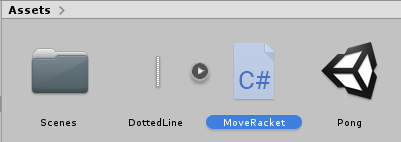下面是我们的脚本当前的样子：

``````using System.Collections;
using UnityEngine;

public class MoveRacket : MonoBehaviour
{
// Start is called before the first frame update
void Start()
{

}

// Update is called once per frame
void Update()
{

}
}
``````

``````using System.Collections;
using UnityEngine;

public class MoveRacket : MonoBehaviour
{
void FixedUpdate()
{

}
}
``````

``````using System.Collections;
using UnityEngine;

public class MoveRacket : MonoBehaviour
{
void FixedUpdate()
{
float v = Input.GetAxisRaw("Vertical");
}
}
``````

``````using System.Collections;
using UnityEngine;

public class MoveRacket : MonoBehaviour
{
void FixedUpdate()
{
float v = Input.GetAxisRaw("Vertical");
GetComponent<Rigidbody2D>().velocity = new Vector2(0, v);
}
}
``````

``````using System.Collections;
using UnityEngine;

public class MoveRacket : MonoBehaviour
{
public float speed = 30;

void FixedUpdate()
{
float v = Input.GetAxisRaw("Vertical");
GetComponent<Rigidbody2D>().velocity = new Vector2(0, v);
}
}
``````

``````using System.Collections;
using UnityEngine;

public class MoveRacket : MonoBehaviour
{
public float speed = 30;
void FixedUpdate()
{
float v = Input.GetAxisRaw("Vertical");
GetComponent<Rigidbody2D>().velocity = new Vector2(0, v) * speed;
}
}
``````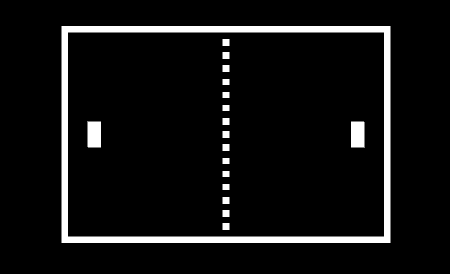只有一个问题，我们还不能分开移动球拍。

### 增加运动轴

``````using System.Collections;
using UnityEngine;

public class MoveRacket : MonoBehaviour
{
public float speed = 30;
public string axis = "Vertical";

void FixedUpdate()
{
float v = Input.GetAxisRaw(axis);
GetComponent<Rigidbody2D>().velocity = new Vector2(0, v) * speed;
}
}
``````

Ball.png

### 球刚体

• 我们不想让它利用地心引力
• 我们希望它有一个很小的质量，这样它就不会在碰撞时推开球拍
• 我们不想让它旋转
• 我们将插值和连续碰撞检测用于精确物理。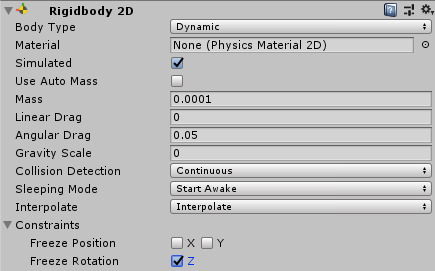注意：这些修改对初学者来说并不是很明显。通常的工作流程是添加一个刚体，测试游戏，然后修改刚体，以防效果太大。

``````using UnityEngine;
using System.Collections;
using System.Collections.Generic;

public class Ball : MonoBehaviour {

// Use this for initialization
void Start () {

}

// Update is called once per frame
void Update () {

}
}
``````

``````using UnityEngine;
using System.Collections;

public class Ball : MonoBehaviour {
public float speed = 30;

void Start() {
// Initial Velocity
GetComponent<Rigidbody2D>().velocity = Vector2.right * speed;
}
}
``````

``````using UnityEngine;
using System.Collections;

public class Ball : MonoBehaviour {
public float speed = 30;

void Start() {
// Initial Velocity
GetComponent<Rigidbody2D>().velocity = Vector2.right * speed;
}

void OnCollisionEnter2D(Collision2D col) {
// Note: 'col' holds the collision information. If the
// Ball collided with a racket, then:
//   col.gameObject is the racket
//   col.transform.position is the racket's position
//   col.collider is the racket's collider
}
}
``````

 1 <- 在球的顶端 0 <- 在球的中间 -1 <- 在球的下面

``````float hitFactor(Vector2 ballPos, Vector2 racketPos,
float racketHeight) {
// ascii art:
// ||  1 <- 在球的顶端
// ||
// ||  0 <- 在球的中间
// ||
// || -1 <- 在球的下面
return (ballPos.y - racketPos.y) / racketHeight;
}
``````

``````void OnCollisionEnter2D(Collision2D col) {
// Note: 'col' holds the collision information. If the
// Ball collided with a racket, then:
//   col.gameObject is the racket
//   col.transform.position is the racket's position
//   col.collider is the racket's collider

// Hit the left Racket?
if (col.gameObject.name == "RacketLeft") {
// Calculate hit Factor
float y = hitFactor(transform.position,
col.transform.position,
col.collider.bounds.size.y);

// Calculate direction, make length=1 via .normalized
Vector2 dir = new Vector2(1, y).normalized;

// Set Velocity with dir * speed
GetComponent<Rigidbody2D>().velocity = dir * speed;
}

// Hit the right Racket?
if (col.gameObject.name == "RacketRight") {
// Calculate hit Factor
float y = hitFactor(transform.position,
col.transform.position,
col.collider.bounds.size.y);

// Calculate direction, make length=1 via .normalized
Vector2 dir = new Vector2(-1, y).normalized;

// Set Velocity with dir * speed
GetComponent<Rigidbody2D>().velocity = dir * speed;
}
}
``````

### 摘要

• 添加一个像顶部显示的拖动效果
• 加上我们都喜欢的古老的乒乓声音
• 分数功能
• 随着时间的推移，提高球的速度
• 增加AI敌人
• 添加菜单和学分屏幕